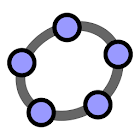GeoGebra Classic

All Android applications categories

All Android games categories# GeoGebra Classic

9.6K 8

8 Users
rating

## Screenshots

Description

GeoGebra Classic joins graphing, geometry, 3D, spreadsheets, computer algebra and probability in one easy-to-use and powerful package. Millions of people around the world use GeoGebra to learn math and science. Join us!

GeoGebra Classic includes the following math tools:
• Graphing: plot functions with sliders and solve equations
• Geometry: create interactive geometric constructions
• 3D Graphing: graph functions, surfaces and many more 3D objects
• Spreadsheet: analyze data and do statistics connected with graphing
• CAS: solve math problems with our powerful computer algebra system
• Probability: visualize parameters and distributions quickly